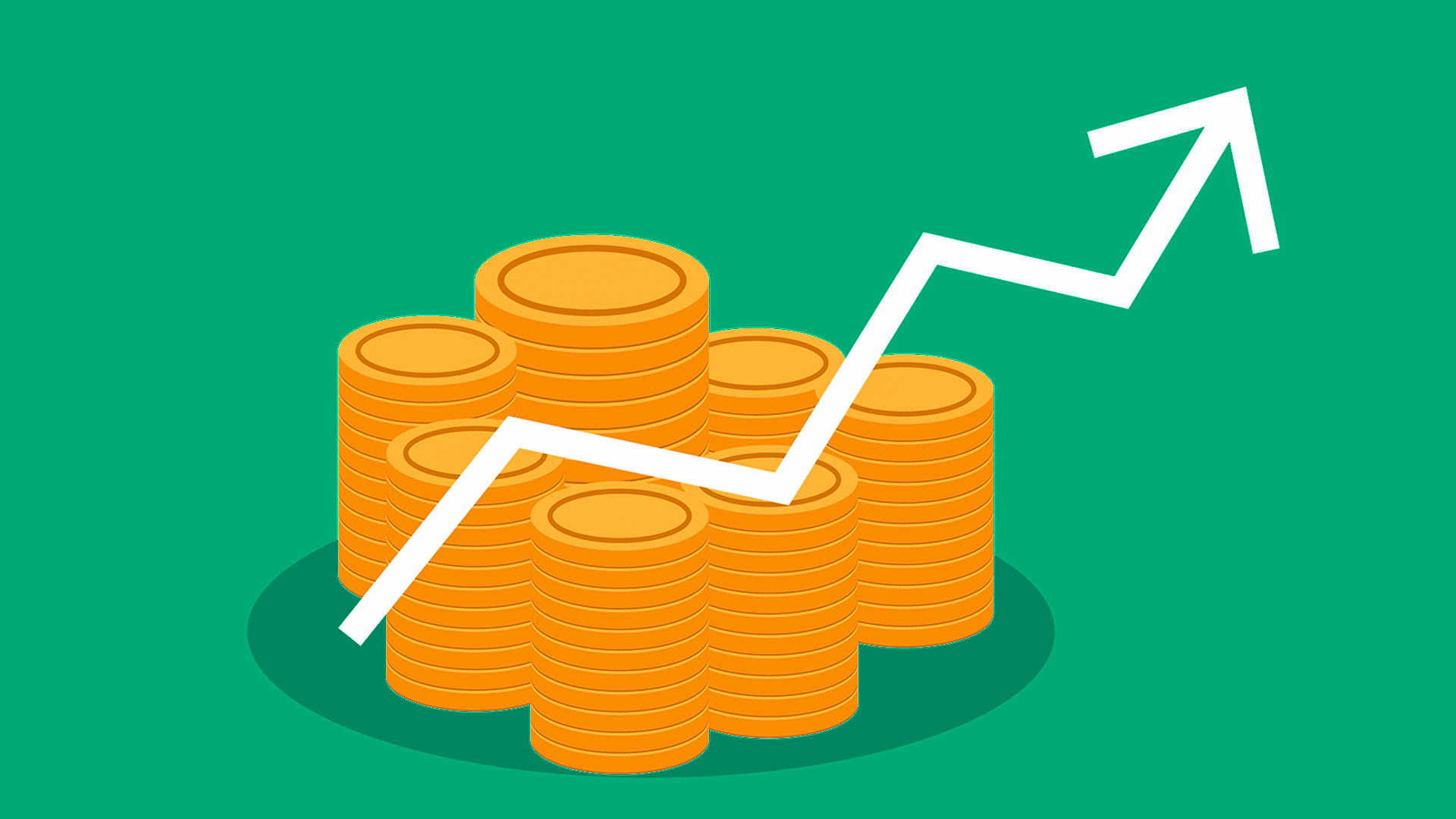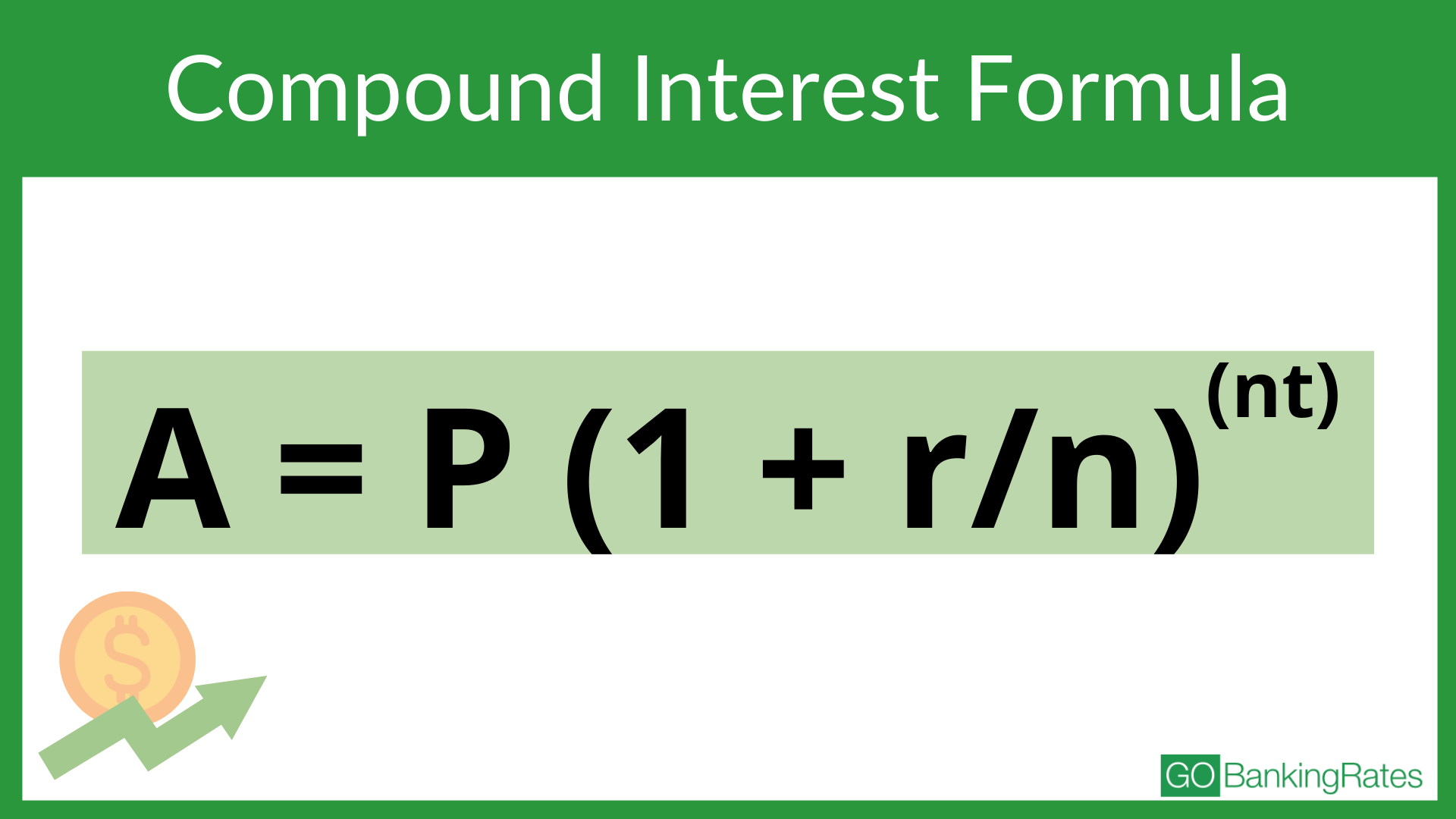# What Is Compound Interest? A Guide To Making It Work for You, Not Against YouA savings account is more than a place to keep your emergency fund. If you leave money in there long enough, it will grow. The money it earns — interest — is free money for you.

Compound interest is the interest paid on the total balance of an account. It includes interest earned on the principal and interest earned on the interest. Lenders don’t give you a compound interest rate or a compound interest loan. Instead, the term refers to the way the interest is added to the account.

Before reading this guide to compound interest, review these terms, which can help you understand compound interest and its effect on your accounts.

### Compound Interest Terms

• Interest: Money paid for the use of another person’s money
• Starting principal: Total amount of money being borrowed, loaned or invested
• Compound frequency: Number of times per year that interest compounds
• Duration: Length of time you will save
• Withdrawals: Money you withdraw from the account

## How To Calculate Compound Interest

You can calculate compound interest either by using an online calculator or the annual compound interest equation. The compound interest in stocks and the compound interest on a loan are calculated using this equation.

### What Is the Compound Interest Formula?

The formula for compound interest is: A = P (1 + r/n)(nt)In the formula:

• P stands for principal — this can be either the amount in your savings account or your loan amount.
• r stands for the annual rate of interest, which should be a decimal.
• t stands for time and is the number of years the principal is deposited or borrowed for.
• A is the accrued amount, which equals principal plus interest.
• n is the number of times the interest is compounded per year.

### What Is Compound Interest and How Does It Work?

For example, say you have \$5,000 in a savings account that earns 2%, compounded yearly. To find out how much your savings will be worth in five years, plug in the numbers to the formula.

Sponsors of• A = P (1 + r/n)(nt)
• A = 5000 (1 +.02/1)^(1(5))
• A = 5000 (1.02)^5
• A = 5000 (1.10408)
• A = 5,520.40

To calculate the compound interest only, use the same formula and subtract the original principal amount. In that case, the compound interest of the above example would be \$520.40 because you subtract the original principal amount of \$5,000 from the calculation.

### Compound Interest on Loans

The same calculation holds true for calculating interest accruing on a debt, such as a credit card balance. Say you hold a credit card debt of \$5,000 with an interest rate of 16% and don’t make any payments. Here is the calculation for how your balance will grow:

• A = P (1 + r/n)(nt)
• A = 5000 (1 + 16/1)^(1(5))
• A = 5000 (1.16)^5
• A = 5000 (2.10)
• A = 10,501.70

In this example, your compound interest totals \$5,501.70, more than doubling the size of your debt in just five years. With simple interest, you would have only accumulated an additional \$4,000 in debt; although still a lot of interest, the power of compounding added 37.5% in interest.

### The Rule of 72: Another Way To Understand What Compound Interest Is and How To Calculate It

To get a rough estimate rather than use a precise formula, follow the “Rule of 72.” Divide 72 by the expected interest rate to determine the number of years it will take to roughly double your money.

For example, earn 10% per year, and you will double your money in about 7.2 years rather than the 10 years you would expect using simple interest (10 years times 10% interest per year equals 100% earnings, or double).

## What Are the Benefits and Drawbacks of Compound Interest?

When you’re saving or investing money, there are plenty of benefits to compound interest. You see similar drawbacks when you’re paying compound interest on money you’ve borrowed.

### Benefits

• Compound interest helps you earn more money in interest over the life of the investment.
• Compound interest earns money quicker than simple interest.
• You don’t have to put away as much money to get to the same goal when you earn compound interest.

### Drawbacks

• You pay more money in interest over the life of the loan when interest is compounded.
• It can take longer to pay off the money you borrow when interest is compounded.

## How To Tell if Interest Is Compounded

One way to understand how compound interest works is to compare what compound interest is versus simple interest. Simple interest means the account earns interest only on the principal. The definition of compound interest is an account earning interest on the principal and all of the interest that has accrued.

### What Is Annual Percentage Yield?

This is the total amount of interest earned on a deposit account per year. APY calculations include compounded interest. APY is often tied to savings and money market accounts or certificates of deposit. You can find details about the APY that applies to an account in a financial institution’s advertisement or in the Truth-in-Lending Disclosure from the bank or lender.

## What Type of Investments Use Compound Interest?

Compound interest is a powerful tool when you’re saving and investing money. Your money can grow exponentially when you have time to leave it in the account for long periods of time.

### Investments That Use Compound Interest:

• CD: A type of savings account in which you keep money for a pre-determined period of time.
• Individual Retirement Account: An account used to save for retirement. Both Roth IRAs and traditional IRAs earn compound interest.
• Savings accounts: An account at banks and credit unions where you deposit money you want to save.
• Savings bond A type of Treasury security citizens can purchase to help the government raise money, which offers a guaranteed return.
• 401(k) An employer-sponsored retirement account.

## What Compound Interest Means To You: Taking Advantage of Compound Interest

When you understand how compound interest works, you can use it to your advantage. You can increase your savings and reduce the amount of money you pay when you borrow from a lender. These tips can help you do just that.

### Save Early

The sooner you start saving, the more time you have to let compound interest work. Here’s what can happen if you put \$10,000 in an account that earns 3% interest that compounds monthly:

• 20 years: \$18,207.55
• 30 years: \$24,568.42
• 40 years: \$33,151.49

### Check APY

Compare accounts. The higher the APY, the more money you stand to earn over time.

Check the APY offered by different banks. Also, check the APY offered on different products at the same bank because rates can vary. Here is the range of APY currently available on CDs through Capital One:

• 6 months: 0.20% APY
• 12 months: 0.30% APY
• 24 months: 0.40% APY
• 60-month: 0.60% APY

Here’s how rates for a 12-month CD compare at four different banks:

• Bank of America: 0.03%
• Capital One: 0.30%
• Citibank: 0.20%
• Marcus by Goldman Sachs: 0.85%

### Know the Compounding Frequency

Interest can compound daily, monthly, semi-annually or annually. For example, if an account earns compound interest annually, the interest is deposited into the account once per year. How often interest compounds can make a dramatic difference in your account balance. Here’s a look at how frequency affects your earnings:

• \$1,000 at 3% compounded daily for 20 years: \$1,822.07
• \$1,000 at 3% compounded monthly for 20 years: \$1,820.75
• \$1,000 at 3% compounded semi-annually for 20 years: \$1,814.02
• \$1,000 at 3% compounded annually for 20 years: \$1,806.11

### Pay Off Your Debt Sooner, Rather Than Later

Compound interest on a loan refers to the interest you pay a lender. When you owe money on a loan or credit card, compound interest works against you. The sooner you pay back the money you borrow, the less it will cost you.

Say, for example, you borrow \$10,000 at 6% interest that compounds daily. If you pay off the loan in five years, you pay 1,603.74 in interest. If you pay off the loan in three years, you pay \$954.27 in interest. That’s a difference of more than \$600 that you could have saved just by paying off your debt more quickly.